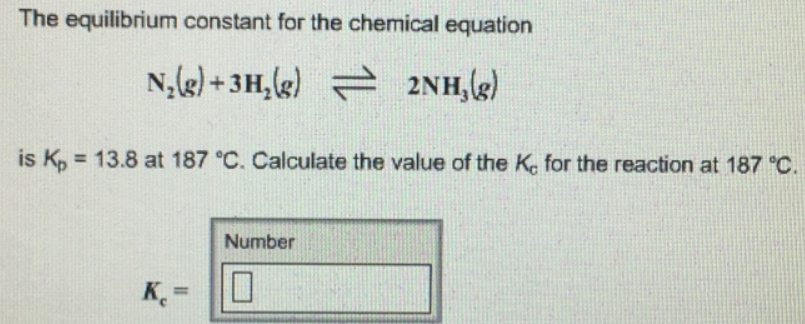# Problem: The equilibrium constant for the chemical equation N2 (g) + 3H2 (g) ⇌ 2NH3 (g)is Kp = 13.8 at 187°C. Calculate the value of the K c for the reaction at 187°C.

###### FREE Expert Solution
99% (478 ratings)###### Problem Details

The equilibrium constant for the chemical equation

N(g) + 3H(g) ⇌ 2NH3 (g)

is Kp = 13.8 at 187°C. Calculate the value of the K c for the reaction at 187°C.